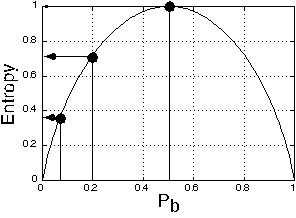Creation of Spatial Structure from Temporal Synchrony

Sang-Hun Lee & Randolph Blake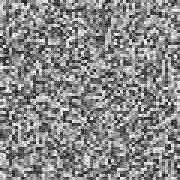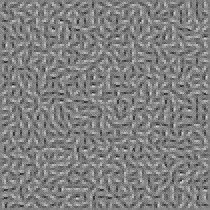This webpage describes and illustrates displays used in our work on spatial structure from temporal structure. Most of the animated demonstrations are QuickTime movies, which can be viewed by clicking on the right-ward pointing arrow at the bottom of the window.

To see demonstrations of spatial grouping from temporal syncrhony without reading background explanation of the displays, select one of the following options:

For a more complete explanation of theory and procedures behind these displays, proceed to the description below.

Our work entails using displays involving variations over time in some property of a visual stimulus, such as color or direction of motion - these time-dependent visual signals constitute "temporal structure." Using information theory, "temporal structure" can be quantified in terms of the predictability of the changes in a given property over time. To begin, let's contrast the difference between deterministic time-varying signals and stochastic time-varying signals, using translational motion as the "carrier" of these signals.

Deterministic Motion and Stochastic Motion

Shown below are two animation sequences, labeled "Deterministic" and "Stochastic" - both consist of a single Gabor patch whose contours (when the movie is activated) move in a direction orthogonal to their orientation. In the "Deterministic" sequence the obliquely oriented contours move back and forth repetitively, with direction (down to the left vs up to the right) shown schematically in the time-line below the animation. These changes in direction of motion over time are completely predictable (i.e., deterministic) - the event has very simple temporal structure. In the "Stochastic" sequence, the contours also move in one of two directions, only here the change in direction of motion is random over time - because of this unpredictability in motion direction, this event has rich temporal structure.

 Deterministic Stochastic

Within the category of stochastic (i.e., random) events we can define and manipulate temporal structure using the concept of entropy. As applied to direction of motion, entropy refers to the predictability (or, conversely, the uncertainty) of motion in a given direction. With low entropy, one direction is highly probable and the other is highly improbable (where "probable" refers to the confidence with which at time n one can predict the direction of motion at time n + 1). With high entropy, two directions of motion are more nearly equal in probability and, therefore, we are highly uncertain about the direction of motion from moment to moment. Examples of low, medium and high entropy motion sequences can be seen by clicking here; more details on entropy appear at the end of this webpage.

Correlation of temporal structure among multiple visual signals

Now consider a condition involving two, spatially separated Gabor patches whose contours move in directions orthogonal to their orientation. (For this example, the two motion signals have maximum entropy - the probability of the two orthogonal directions is 0.50). If the changes in direction of motion in one Gabor patch are completely independent of the changes in direction of motion in the other Gabor patch, we say the two are uncorrelated. At the other extreme, if both Gabor gratings change direction motion in perfect synchrony, we say they are 100% correlated. Click here to see an animations illustrating three different levels of correlation. It must be stressed that "correlation" in these displays refers only to the degree to which the changes in direction of motion occur simultaneously (i.e., the extent to which their point processes resemble one another), not to the directions of motion themselves.

Now imagine a large array of small Gabor patches, with the orientation of each Gabor being random with respect to the others. As described above, the contours within each patch move in one of the two directions orthogonal to the orientation of its contours. At random points in time, the direction of motion reverses, meaning that each Gabor patch follows its own point process. Now imagine that we define a "virtual rectangle" somewhere within this array and stipulate that the average correlation of the point processes of Gabor patches within this "rectangle" be a particular value. (Average correlation is, in fact, indexed by computing the correlations between every possible pair of point processes within this rectangle and then taking the average of those correlations.) Point processes for Gabor patches outside this "rectangle" are uncorrelated (meaning that their changes in direction of motion occur independently). The schematic below shows the point processes for two arbitrarily selected Gabor patches within the virtual rectangle and two point processes for Gabor patches outside the rectangle.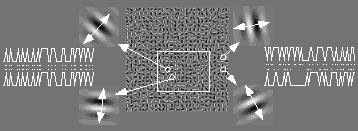We vary the aspect ratio (height/width) of this rectangular region, making it either vertical or horizontal, and we vary the average correlation of the point processes within the virtual region. The actual location of the "virtual rectangle" within the array varies randomly from trial to trial. When correlation is high, the "rectangle" stands out conspicuously from the background, and one can judge the axis of elongation of the "rectangle" even for aspect ratios near unity. Click here to see animation sequences that illustrate how the vividness of the rectangle depends on correlation. We must stress that in these displays there is no spatial structure defined by luminance, contrast, orientation, or direction of motion. It is only the temporal synchrony of direction change that sets off one region from another. (Video frame-rate influences the vividness of figure/ground segregation - if you have trouble seeing the rectangle, try moving the slider manually at different rates.)

Point Process

Shown below are four sets of point processes, each associated with a given "average" correlation among Gabor elements. Within each set, a row of dots denotes the point in time at which the direction of motion for that Gabor reversed. For sake of example, we are showing 10 Gabor point processes within each set; shown at the right of each set is the average correlation value for that stimulus.Strucure From Rotational Motion

We have also utilized rotational motion to generate spatial structure from temporal structure. In these displays each set of oriented contours rotates clockwise or counterclockwise, changing direction of rotation randomly over time. The changes over time in direction of rotation of each Gabor patch generate a point process, and those point processes can be correlated to varying degrees within a virtual region of the display. Click here to see an example of 100% correlation.

Spatial Structure from Random Luminance Modulation

Finally, we have also created displays in which temporal structure is created by random changes in the luminance of small pixels. Imagine a 2-dimensional array of gray-scale pixels each of which varies randomly in luminance over time. Looking at the figure below, the middle of the picture shows one frame from such a sequence. The two time series plotted on left and the two plotted on the right show changes in luminance over time of four pixels in this display. For the two pixels within the virtual rectangular region, changes in luminance are coincident in time, as shown by the correlation between their two point processes (the series of small dots). For the two pixels outside the virtual rectangle, changes in luminance are uncorrelated in time. Note that there is absolutely no "shape" information in any given frame of such a sequence - each and every pixel has its own, randomly determined luminance. And when each pixel follows an independent, random pattern of change over time, there is no spatial structure whatsoever in space or in time. The display simply looks like an array of flickering pixels, with no spatial structure - this is the animation you saw at the beginning of this webpage. The average correlation among point processes describing the change in luminance of each and every pixel is zero.But suppose some pixels within a "virtual region" of the array change in luminance at the same time, while those outside of this region change entirely randomly. Note that the pixels within the virtual area do not have the same luminance values nor do their luminance changes follow the same pattern of increases and decreases - individual pixel values remain entirely random in luminance. However, the temporal synchrony of their changes relative to those in the surround does represent a form of "common fate" (in the Gestalt sense of that phrase). And it is possible to segregate this virtual area from its background based solely on this temporal synchrony. Click here to see animations illustrating spatial structure from random changes in luminance.

Information Theory

We can define temporal structure in these displays using information theory. Consider the information available in a simple motion display in which a patch of oriented contours (a Gabor element) moves in one of two orthogonal directions, with the probability of a change in direction determined by a random time-series. The two alternative directions of motion can be construed as a series of messages "a" and "b".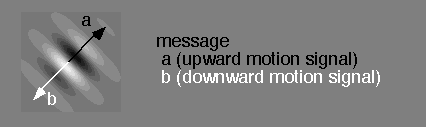We can plot that series of messages in the manner shown below.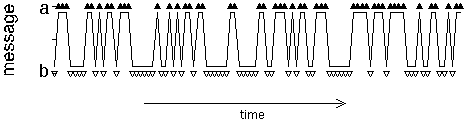The "average" information contained in this series of messages is given by the quantity Hav:

Hav = -1(Pa*log2(Pa) + Pb*log2(Pb))

and the average duration of these two messages is given by Tav:

Tav = Pa*Ta + Pb*Tb

With these two quantities, we can express the information flow rate:

Information flow rate = Hav / Tav

Entropy

Because the motion in each of the Gabor patches is stochastic in nature, we can employ the concept of entropy to define the average amount of information conveyed by each Gabor. Specifically, each Gabor patch can be construed as a carrier that broadcasts either of two, mutually exclusive messages, corresponding to the two possible directions of motion for the contours defining that Gabor (directions "a" and "b" in the example shown below). We can manipulate the entropy of this visual signal by varying the probabilities of these two messages. When the probability of one message is high (and, therefore, the probability of the other is low), the associated entropy is low; when the two messages are equally probable, entropy is high. The figure below shows three different examples of entropy for a single Gabor patch. Entropy is expressed in "frame" units since direction of motion was independently determined for every new frame of the animation.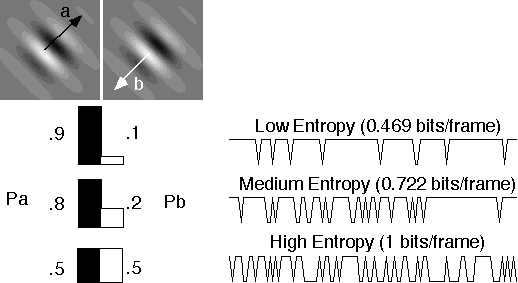The graph below shows how entropy varies with probability for these "two-state" messages (i.e., messages that assume one of two values with probabilities Pa and Pb).